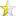Jan 14, 2008

# Tired of shifting bits?I've looked at one too many pieces of source code that uses bit shifting along with bitwise ANDs to extract a value from a larger data size (for example, an IP address from a 32-bit integer).

This is error-prone. I'm going to show you an easier way, using a C "union".

A C union is just a simple way to refer to the same memory address using more than one symbolic label.

Okay, its not "that" simple, so here's a slightly simpler explanation. You know when you assign an name to a variable, what you're really doing is giving a piece of memory a symbol, a (symbolic) name you can use to refer to it. So when we write, for example, int x, what we're really doing is giving a 4-byte (on 32-bit machines) location of ram a label, "x", that we can refer to in our code.

A union takes this a step further, by allowing us to refer to the same memory location by 2 or more names, and access them as if they're 2 or more data types.

What I'm going to do is create a structure that will allow me to stuff a 32-bit integer into a location, and then extract the individual byte values from the same location, or alternately, write 4 individual bytes, and get the 32-bit integer value, all without any shifts or logical ands.

I do this in 2 steps:
1. declare a structure of 4 bytes (4 unsigned chars, to be exact), that I can address as b0, b1, b2 and b3.[/li]
2. declare a union that allows this 4 byte structure to overlap the same memory address as an unsigned 4-byte integer;[/li]
Here's the code:

 ``123456789101112131415`` ``````#include #include typedef struct packed_int { unsigned char b0; unsigned char b1; unsigned char b2; unsigned char b3; } packed_int; typedef union { unsigned int i; packed_int b; } packed; ``````

Okay, now I just need some code to "exercise" these structures, to show you that they actually do what I say, without any fancy bit-shifting in the source ... (note that I'm not including code to account for "endian-ness" - this would be conditionally included by using a compile-time constant, but its left out here for the sake of clarity).

 ``1234567891011121314151617181920212223242526272829303132`` `````` int main(int argc, char* argv[], char* env[]) { packed v; /* this is my "dual-purpose" 32-bit memory location */ v.i = 3232235881UL; /* I assign it an internet address in 32-bit format */ /* next, I'll print out the individual bytes */ printf("the bytes are %d, %d, %d, %d\n", v.b.b0, v.b.b1, v.b.b2, v.b.b3); /* and just to prove that the 32-bit integer is still there ... print it out too */ printf("the value is %u\n", v.i); /* just for the heck of it, increment the 32-bit integer */ v.i++; printf("after v.i++, the bytes are %d, %d, %d, %d\n", v.b.b0, v.b.b1, v.b.b2, v.b.b3); /* now do the reverse, assign 70.80.90.100 as an ip address */ v.b.b0 = 70; v.b.b1 = 80; v.b.b2 = 90; v.b.b3 = 100; /* .. and extract the 32-bit integer value */ printf("the value is %u\n", v.i); /* show that 70.80.90.100 is really what we put in there */ printf("the bytes are %d, %d, %d, %d\n", v.b.b0, v.b.b1, v.b.b2, v.b.b3); /* ok, we're done here */ return EXIT_SUCCESS; } ``````

Now, isn't that a lot easier than creating some freak-show macros to do bit manipulation? BTW, the same trick works for point_t, rect_t, time_t, 64, 128, and 256 bit values, as well as individual bits.

In a future post, I'll show you how to write code to select individual bits without bothering with bitmasks.

my original article source: http://trolltalk.com/index.php?name=News&file=article&sid=2]]